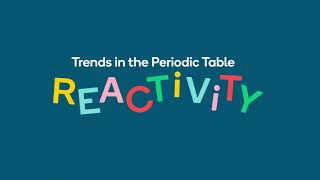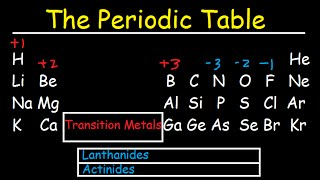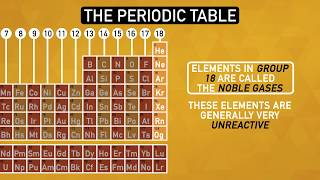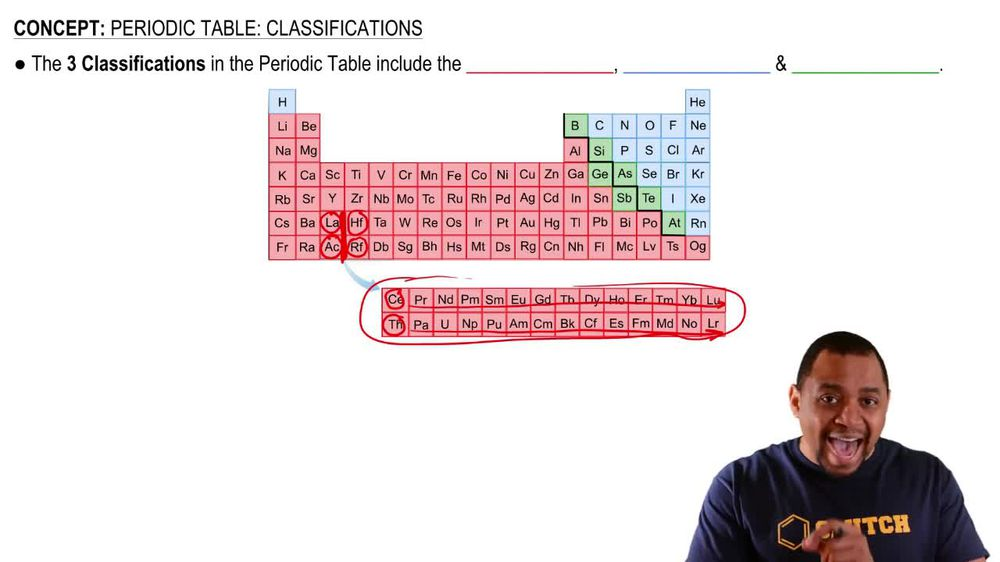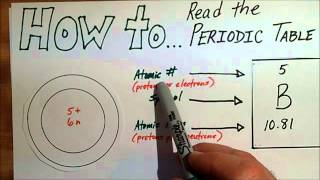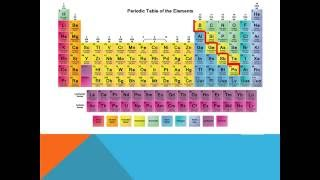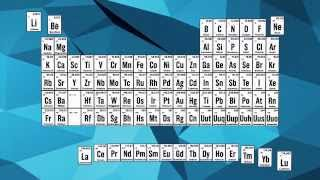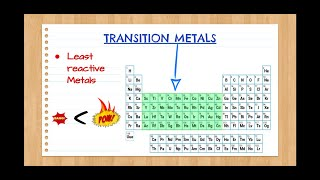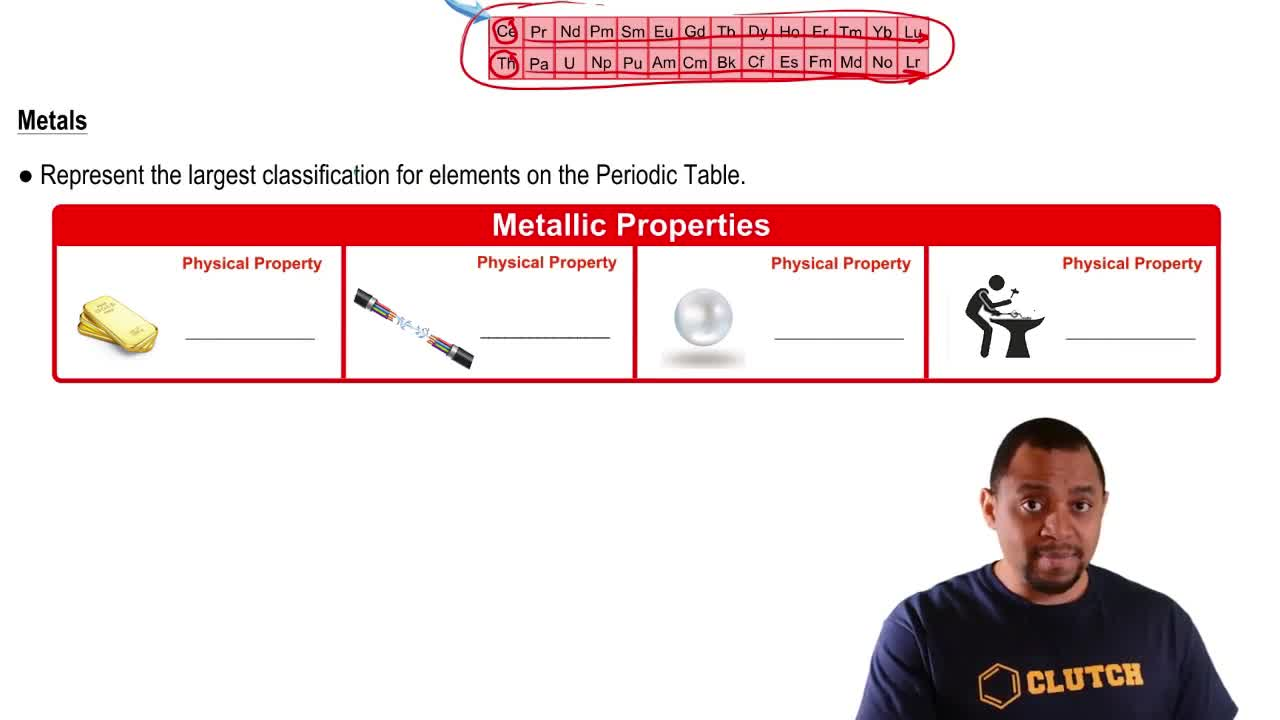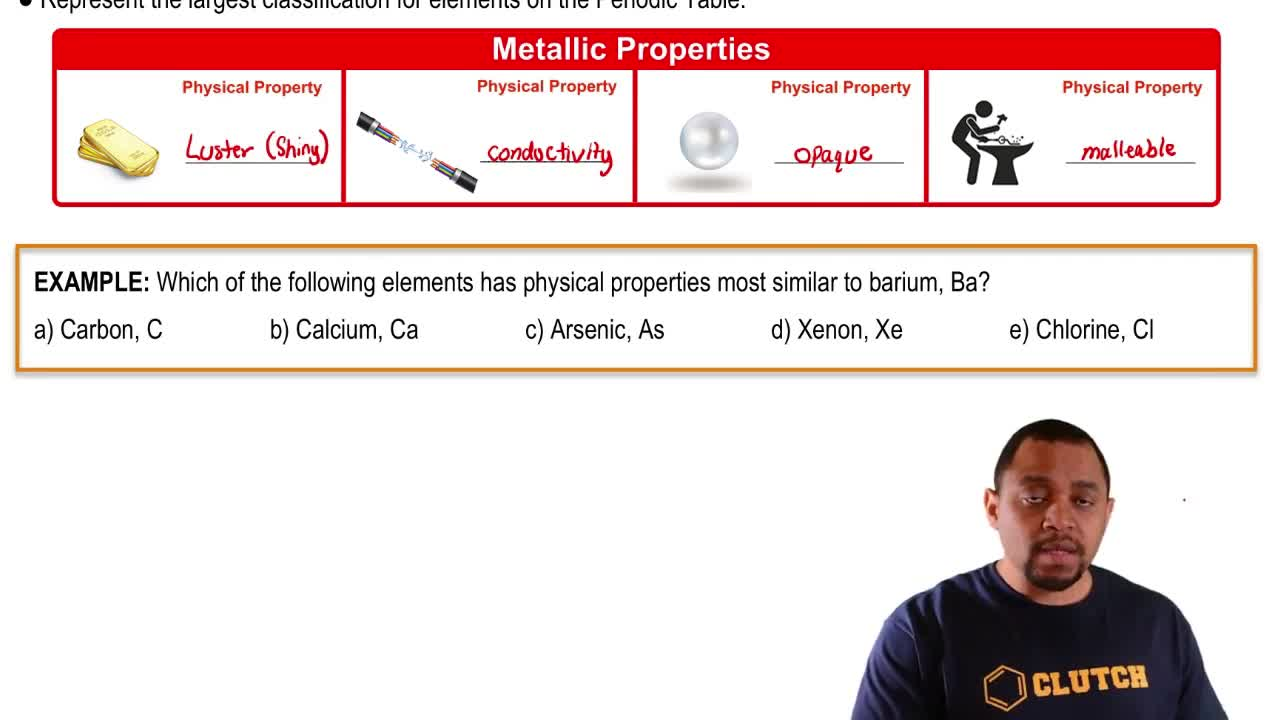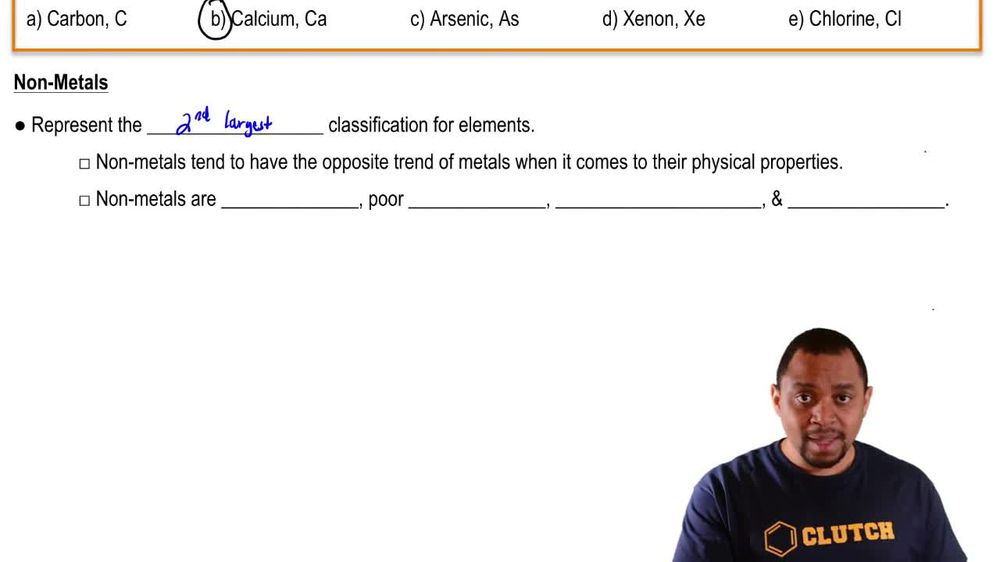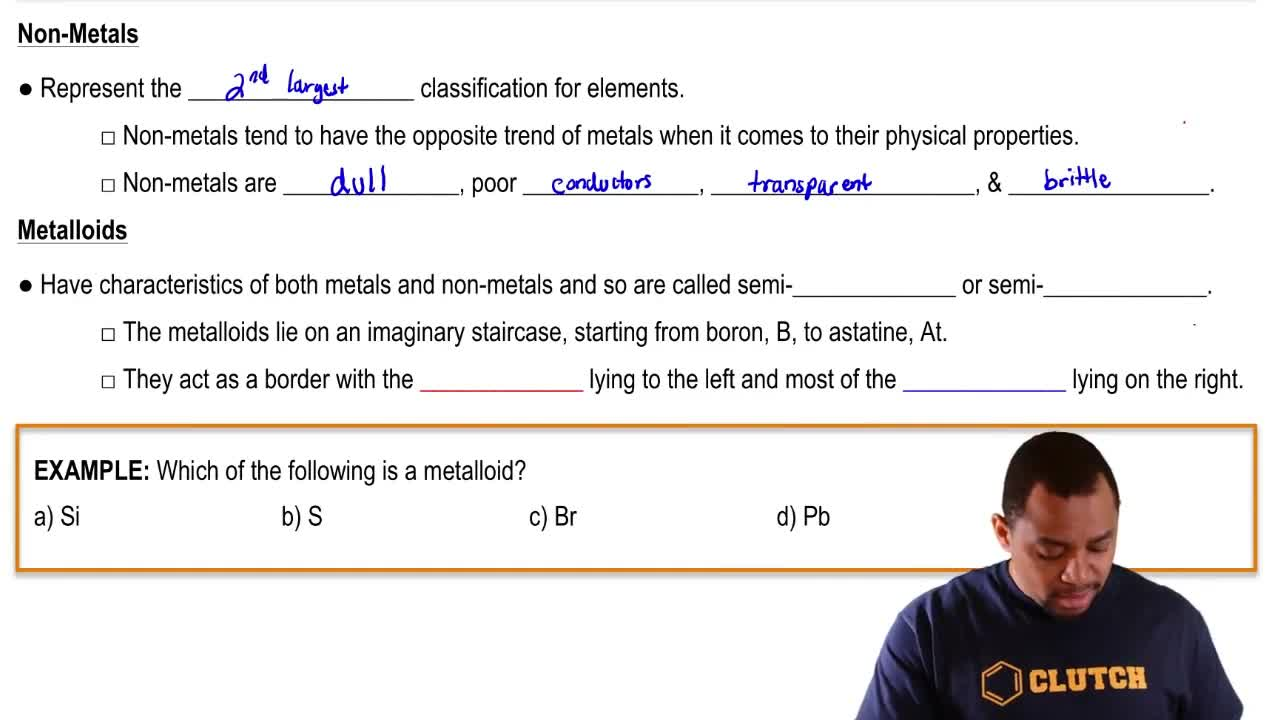Start typing, then use the up and down arrows to select an option from the list.
1. 2. Atoms & Elements2. Periodic Table: Classifications
Problem

# Where in the periodic table are the metallic elements found? Where are the nonmetallic elements found?

Relevant Solution1m
Play a video:
Hi everyone for this problem. It reads where is the location of the metallic and non metallic elements on the periodic table? All right. So we want to know which ones are metals and non metals. And we have a color coded periodic table here. So let's go ahead and decipher what are color codes mean? So the red color coded elements represent non metals. Okay, So we see that we have the hydrogen to the left, at the top left corner and then on the right are the rest of our non metals. Next we have blue. The blue here represents metals. So we see all of our medals and green represents metal lloyds. Okay, so the question asked for the location of the metals and non metals. And so our answer here is the non metals are shaded red and the metals are shaded blue. So that's it for this problem. I hope this was helpful.